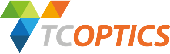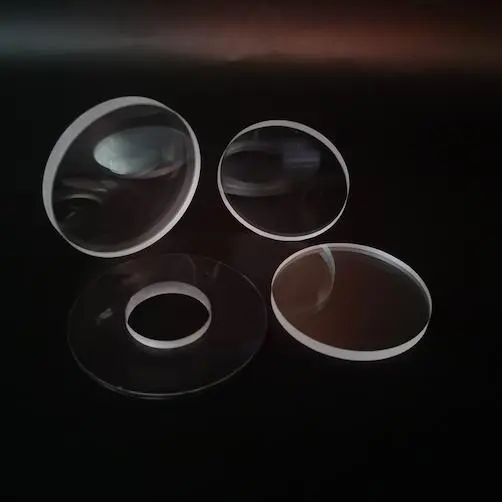﻿ Basic Introduction to Spherical Mirrors - TianCheng Optics CO., Ltd.# Basic Introduction to Spherical Mirrors## Basic concepts of spherical mirrors

• Vertex: The center point O of the mirror is called the vertex of the mirror.

• Center: The center of the sphere C is called the center of the mirror.

• Principal axis: The line connecting the vertex O and the center of the mirror.

• Focus: When a parallel ray of light falls on the mirror, its reflected ray converges to a point on the principal axis. This point is called the focus and is represented by the letter F. The focus of a convex mirror is not the actual convergence point of the light, but the intersection of the extended line of the reflected ray of the light, therefore, the focus of a convex mirror is a virtual focus.

• Focal length: The distance between the focus and the vertex is called the focal length and is represented by the letter f. f=R/2, that is, the focal length of a concave mirror is equal to half the radius of the sphere.

## Different Types of spherical mirrors

Spherical mirrors are divided into two types: convex and concave mirrors.

• Concave mirror: A spherical mirror in which the inner surface of the sphere is used as the reflecting surface is called a concave mirror.

• Convex mirror: A spherical mirror in which the outer surface of the sphere is used as the reflecting surface is called a convex mirror.

## Optical characteristics of spherical mirrors

### Optical characteristics of concave mirrors:

• The reflection phenomenon on the concave mirror follows the law of reflection.

• The light that is parallel to the principal axis reflects off the concave mirror and converges to the focus. The focus on the concave mirror is the actual convergence point of the light, therefore, it is a real focus.

• The concave mirror converges the light and the smaller the focal length, the stronger the convergence ability.

• Four special rays: the light that is parallel to the principal axis reflects off the concave mirror and converges to the focus; the incident light passing through the focus reflects off the mirror and becomes parallel to the principal axis; the incident light passing the center of the sphere is reflected along the same path; the incident light passing through the vertex and its reflected light are symmetric about the principal axis.

### Optical characteristics of convex mirrors:

• The reflection phenomenon on the convex mirror follows the law of reflection.

• The focus of the convex mirror is a virtual focus.

• The convex mirror diverges the light and the smaller the focal length, the stronger the divergence ability.

• Four special rays (similar to concave mirrors).

## Applications of spherical mirrors

### Applications of concave mirrors:

• Use the converging effect of a concave mirror on light rays: solar furnaces.

• Use the light passing through the focus and reflecting off the mirror to become parallel light: searchlights, flashlights, and the headlights of various vehicles.

### Applications of convex mirrors:

• Use the diverging effect of a convex mirror on light rays: car rearview mirrors.

TC Optics is a manufacturer of optical components. We are dedicated to producing windows, spherical lenses, aspherical lenses, prisms, filters, and mirrors. Please feel free to contact us for inquiries.TCOPTICS, Your Professional Optics Manufacturing Company
NEWS
• 18686471870

• 86-431-89851801